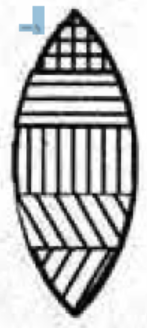A convex lens is made of five different materials as shown in the figure. How many images does it form? Why?

# A convex lens is made of five different materials as shown in the figure. How many images does it form? Why?Fill Out the Form for Expert Academic Guidance!l

+91

Live ClassesBooksTest SeriesSelf Learning

Verify OTP Code (required)

### Solution:

A convex lens made of five different materials will form 4 different images because it will have five different refractive indices. Due to different refractive indices, the materials will have different focal lengths. Hence different images will be formed at different places according to the focal length of the respective material.

Hence the material will form one image for each refractive index or focal length.

## Related content

 Difference Between Mass and Weight Differences & Comparisons Articles in Physics Important Topic of Physics: Reynolds Number Distance Speed Time Formula Refractive Index Formula Mass Formula Electric Current Formula Electric Power Formula Resistivity Formula Weight Formula+91

Live ClassesBooksTest SeriesSelf Learning

Verify OTP Code (required)